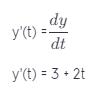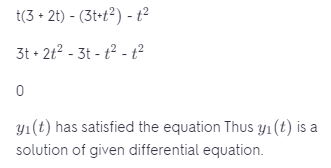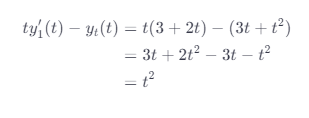# Verify That Each Given Function Is A Solution Of The Differential Equation

We thoroughly check each answer to a question to provide you with the most correct answers. Found a mistake? Let us know about it through the REPORT button at the bottom of the page.

Verify that each given function is a solution of the differential equation. ty’−y=t2; y=3t+t2

We have differentiated y(t)Plug the values of y(t) and y'(t) in the given differential equation.y is a solution of a given differential equation.